# MLJAR Automated Machine Learning for HumansDocumentation: https://supervised.mljar.com/# Automated Machine Learning

The `mljar-supervised` is an Automated Machine Learning Python package that works with tabular data. It is designed to save time for a data scientist. It abstracts the common way to preprocess the data, construct the machine learning models, and perform hyper-parameters tuning to find the best model 🏆. It is no black-box as you can see exactly how the ML pipeline is constructed (with a detailed Markdown report for each ML model).

The `mljar-supervised` will help you with:

• explaining and understanding your data (Automatic Exploratory Data Analysis),
• trying many different machine learning models (Algorithm Selection and Hyper-Parameters tuning),
• creating Markdown reports from analysis with details about all models (Automatic-Documentation),

It has four built-in modes of work:

• `Explain` mode, which is ideal for explaining and understanding the data, with many data explanations, like decision trees visualization, linear models coefficients display, permutation importances and SHAP explanations of data,
• `Perform` for building ML pipelines to use in production,
• `Compete` mode that trains highly-tuned ML models with ensembling and stacking, with a purpose to use in ML competitions.
• `Optuna` mode that can be used to search for highly-tuned ML models, should be used when the performance is the most important, and computation time is not limited (it is available from version `0.10.0`)

Of course, you can further customize the details of each `mode` to meet the requirements.We are working on Excel Add-in for Machine Learning. You can train ML models without leaving WorkSheet. Model training is done locally on your machine (no cloud). You can train models with MLJAR AutoML or single models (manual hyperparameter selection).

Interested? Please fill out the form, and we will inform you when it will be available.

## What's good in it?

• It is using many algorithms: `Baseline`, `Linear`, `Random Forest`, `Extra Trees`, `LightGBM`, `Xgboost`, `CatBoost`, `Neural Networks`, and `Nearest Neighbors`.
• It can compute Ensemble based on greedy algorithm from Caruana paper.
• It can stack models to build level 2 ensemble (available in `Compete` mode or after setting `stack_models` parameter).
• It can do features preprocessing, like: missing values imputation and converting categoricals. What is more, it can also handle target values preprocessing.
• It can do advanced features engineering, like: Golden Features, Features Selection, Text and Time Transformations.
• It can tune hyper-parameters with `not-so-random-search` algorithm (random-search over defined set of values) and hill climbing to fine-tune final models.
• It can compute the `Baseline` for your data. That you will know if you need Machine Learning or not!
• It has extensive explanations. This package is training simple `Decision Trees` with `max_depth <= 5`, so you can easily visualize them with amazing dtreeviz to better understand your data.
• The `mljar-supervised` is using simple linear regression and include its coefficients in the summary report, so you can check which features are used the most in the linear model.
• It cares about explainability of models: for every algorithm, the feature importance is computed based on permutation. Additionally, for every algorithm the SHAP explanations are computed: feature importance, dependence plots, and decision plots (explanations can be switched off with `explain_level` parameter).
• There is automatic documentation for every ML experiment run with AutoML. The `mljar-supervised` creates markdown reports from AutoML training full of ML details, metrics and charts.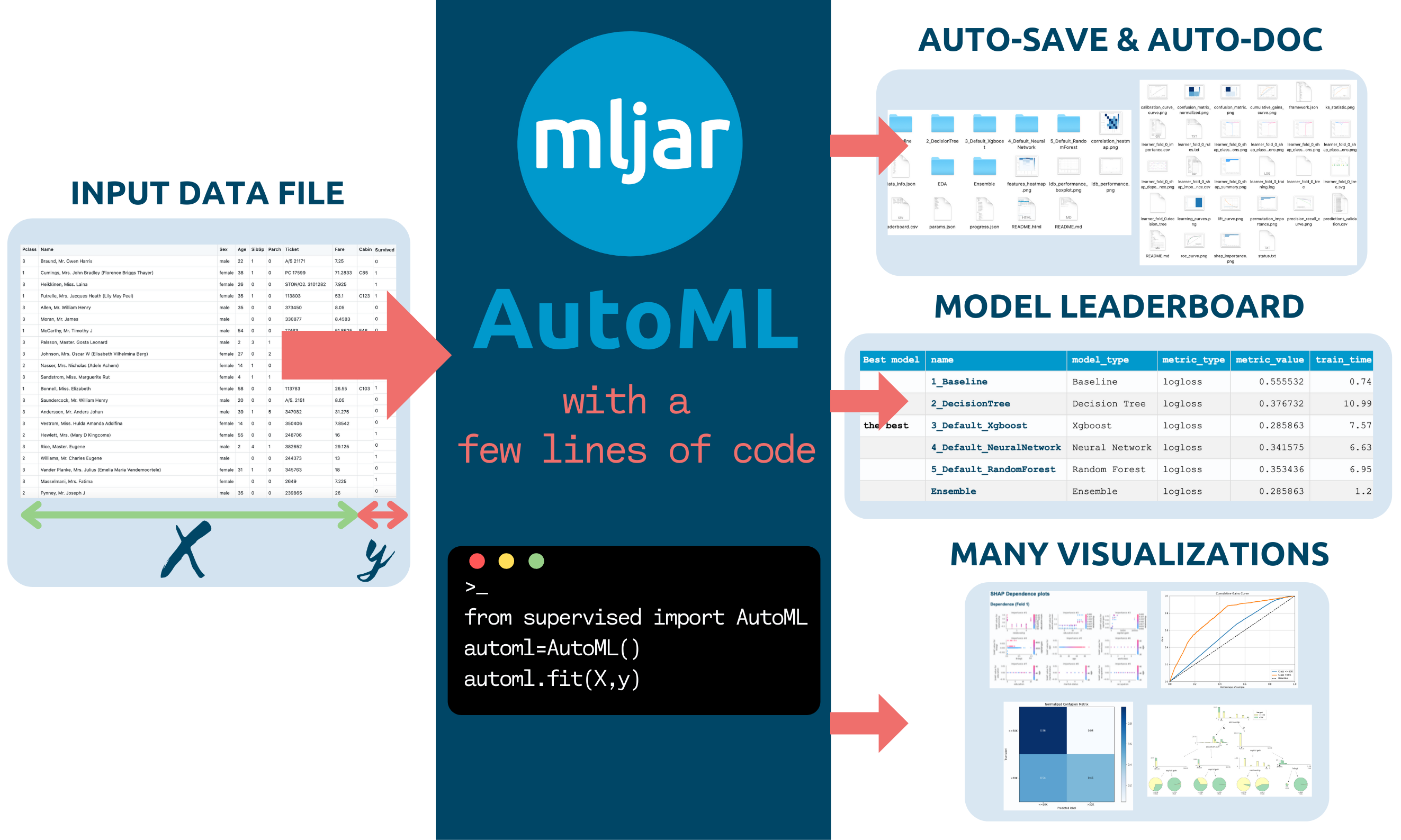# Automatic Documentation

## The AutoML Report

The report from running AutoML will contain the table with infomation about each model score and time needed to train the model. For each model there is a link, which you can click to see model's details. The performance of all ML models is presented as scatter and box plots so you can visually inspect which algorithms perform the best 🏆.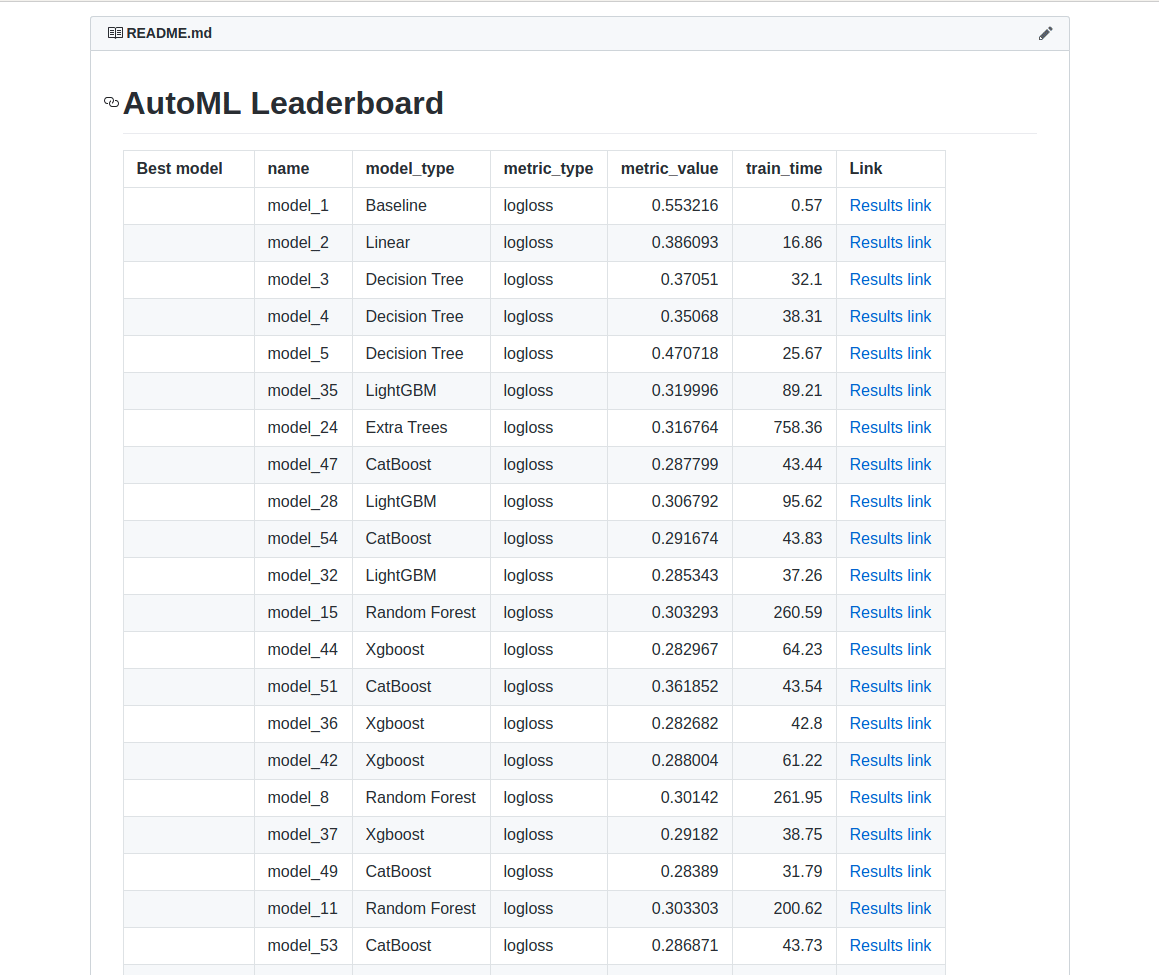## The `Decision Tree` Report

The example for `Decision Tree` summary with trees visualization. For classification tasks additional metrics are provided:

• confusion matrix
• threshold (optimized in the case of binary classification task)
• F1 score
• Accuracy
• Precision, Recall, MCC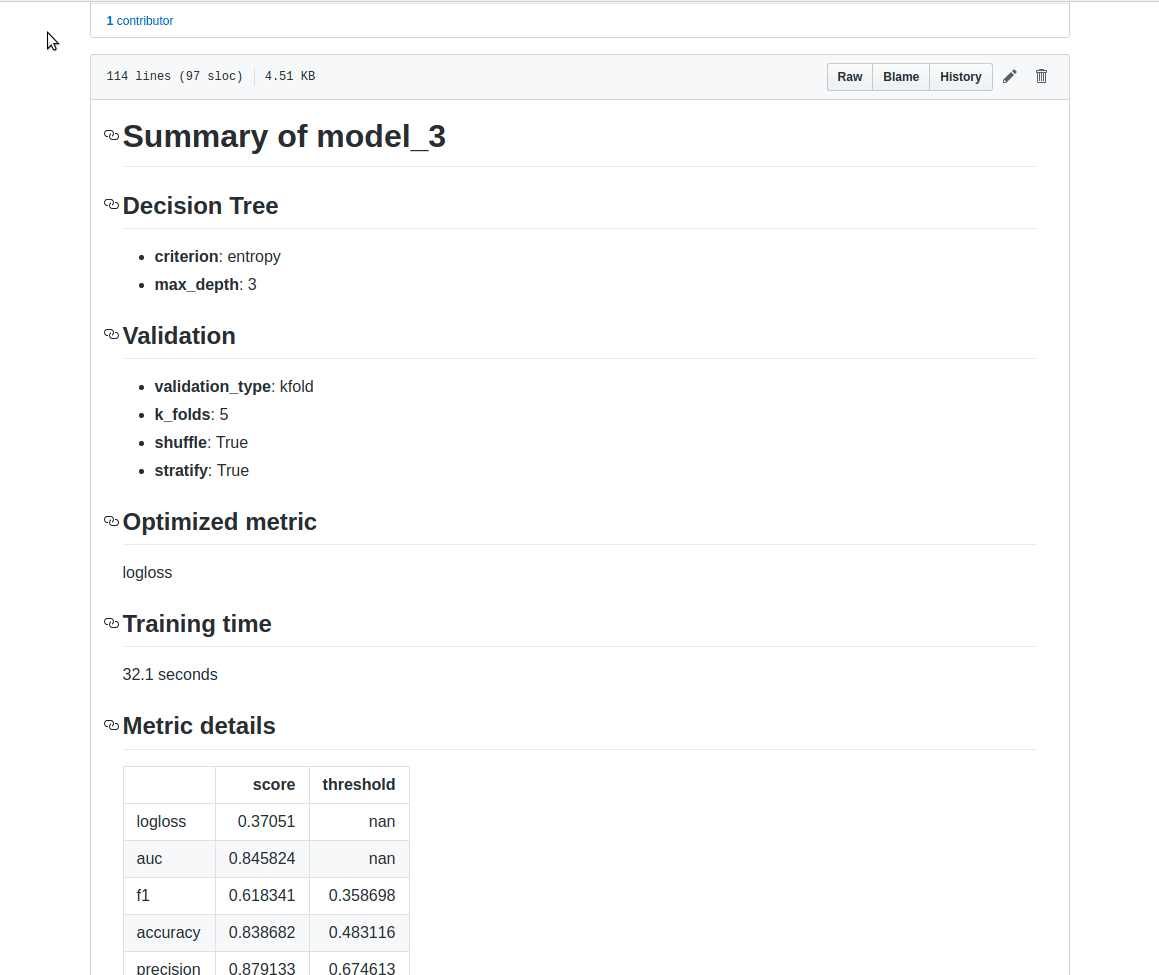## The `LightGBM` Report

The example for `LightGBM` summary: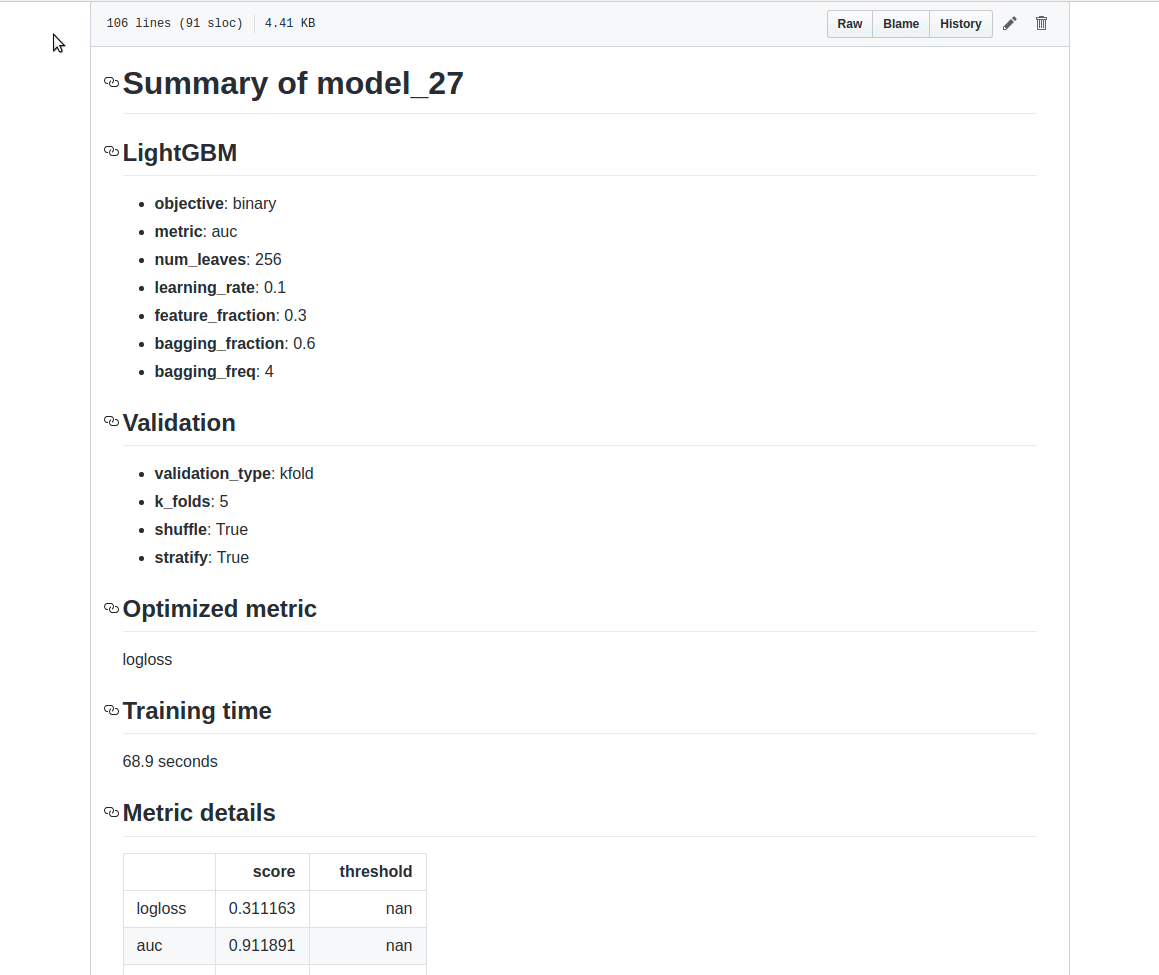## Available Modes

In the docs you can find details about AutoML modes are presented in the table .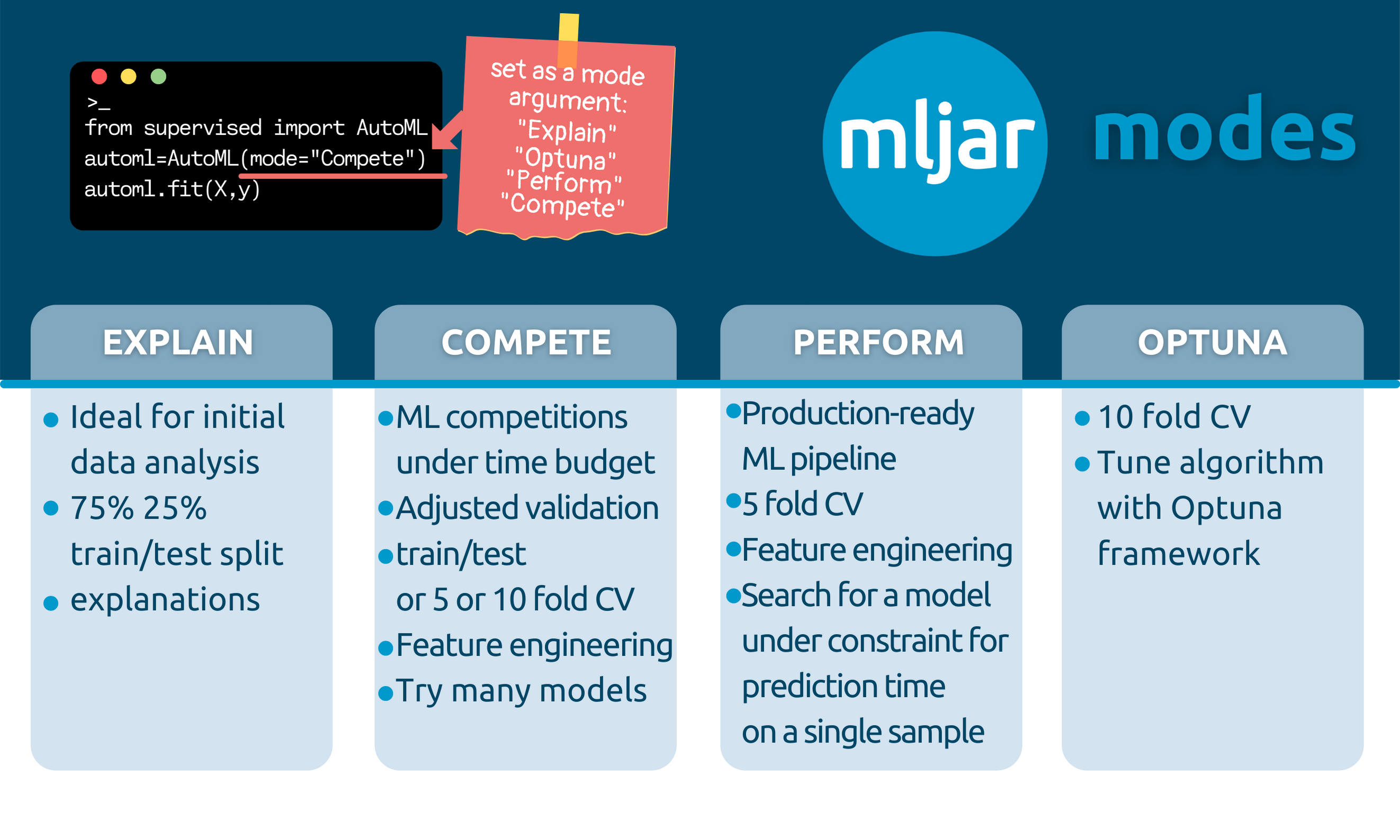### Explain

``````automl = AutoML(mode="Explain")
``````

It is aimed to be used when the user wants to explain and understand the data.

• It is using 75%/25% train/test split.
• It is using: `Baseline`, `Linear`, `Decision Tree`, `Random Forest`, `Xgboost`, `Neural Network` algorithms and ensemble.
• It has full explanations: learning curves, importance plots, and SHAP plots.

### Perform

``````automl = AutoML(mode="Perform")
``````

It should be used when the user wants to train a model that will be used in real-life use cases.

• It is using 5-fold CV.
• It is using: `Linear`, `Random Forest`, `LightGBM`, `Xgboost`, `CatBoost` and `Neural Network`. It uses ensembling.
• It has learning curves and importance plots in reports.

### Compete

``````automl = AutoML(mode="Compete")
``````

It should be used for machine learning competitions.

• It adapts the validation strategy depending on dataset size and `total_time_limit`. It can be: train/test split (80/20), 5-fold CV or 10-fold CV.
• It is using: `Linear`, `Decision Tree`, `Random Forest`, `Extra Trees`, `LightGBM`, `Xgboost`, `CatBoost`, `Neural Network` and `Nearest Neighbors`. It uses ensemble and stacking.
• It has only learning curves in the reports.

### Optuna

``````automl = AutoML(mode="Optuna", optuna_time_budget=3600)
``````

It should be used when the performance is the most important and time is not limited.

• It is using 10-fold CV
• It is using: `Random Forest`, `Extra Trees`, `LightGBM`, `Xgboost`, and `CatBoost`. Those algorithms are tuned by `Optuna` framework for `optuna_time_budget` seconds, each. Algorithms are tuned with original data, without advanced feature engineering.
• It is using advanced feature engineering, stacking and ensembling. The hyperparameters found for original data are reused with those steps.
• It produces learning curves in the reports.

## How to save and load AutoML?

All models in the AutoML are saved and loaded automatically. No need to call `save()` or `load()`.

### Example:

#### Train AutoML

``````automl = AutoML(results_path="AutoML_classifier")
automl.fit(X, y)
``````

You will have all models saved in the `AutoML_classifier` directory. Each model will have a separate directory with the `README.md` file with all details from the training.

#### Compute predictions

``````automl = AutoML(results_path="AutoML_classifier")
automl.predict(X)
``````

The AutoML automatically loads models from the `results_path` directory. If you will call `fit()` on already trained AutoML then you will get a warning message that AutoML is already fitted.

### Why do you automatically save all models?

All models are automatically saved to be able to restore the training after interruption. For example, you are training AutoML for 48 hours, and after 47 hours there is some unexpected interruption. In MLJAR AutoML you just call the same training code after the interruption and AutoML reloads already trained models and finish the training.

## Supported evaluation metrics (`eval_metric` argument in `AutoML()`)

• for binary classification: `logloss`, `auc`, `f1`, `average_precision`, `accuracy`- default is `logloss`
• for mutliclass classification: `logloss`, `f1`, `accuracy` - default is `logloss`
• for regression: `rmse`, `mse`, `mae`, `r2`, `mape`, `spearman`, `pearson` - default is `rmse`

If you don't find `eval_metric` that you need, please add a new issue. We will add it.

# Examples

## 👉 Binary Classification Example

There is a simple interface available with `fit` and `predict` methods.

``````import pandas as pd
from sklearn.model_selection import train_test_split
from supervised.automl import AutoML

skipinitialspace=True,
)
X_train, X_test, y_train, y_test = train_test_split(
df[df.columns[:-1]], df["income"], test_size=0.25
)

automl = AutoML()
automl.fit(X_train, y_train)

predictions = automl.predict(X_test)
``````

AutoML `fit` will print:

``````Create directory AutoML_1
AutoML task to be solved: binary_classification
AutoML will use algorithms: ['Baseline', 'Linear', 'Decision Tree', 'Random Forest', 'Xgboost', 'Neural Network']
AutoML will optimize for metric: logloss
1_Baseline final logloss 0.5519845471086654 time 0.08 seconds
2_DecisionTree final logloss 0.3655910192804364 time 10.28 seconds
3_Linear final logloss 0.38139916864708445 time 3.19 seconds
4_Default_RandomForest final logloss 0.2975204390214936 time 79.19 seconds
5_Default_Xgboost final logloss 0.2731086827200411 time 5.17 seconds
6_Default_NeuralNetwork final logloss 0.319812276905242 time 21.19 seconds
Ensemble final logloss 0.2731086821194617 time 1.43 seconds
``````
• the AutoML results in Markdown report
• the Xgboost Markdown report, please take a look at amazing dependence plots produced by SHAP package 💖
• the Decision Tree Markdown report, please take a look at beautiful tree visualization ✨
• the Logistic Regression Markdown report, please take a look at coefficients table, and you can compare the SHAP plots between (Xgboost, Decision Tree and Logistic Regression) ☕️

## 👉 Multi-Class Classification Example

The example code for classification of the optical recognition of handwritten digits dataset. Running this code in less than 30 minutes will result in test accuracy ~98%.

``````import pandas as pd
# scikit learn utilites
from sklearn.metrics import accuracy_score
from sklearn.model_selection import train_test_split
# mljar-supervised package
from supervised.automl import AutoML

X_train, X_test, y_train, y_test = train_test_split(
pd.DataFrame(digits.data), digits.target, stratify=digits.target, test_size=0.25,
random_state=123
)

# train models with AutoML
automl = AutoML(mode="Perform")
automl.fit(X_train, y_train)

# compute the accuracy on test data
predictions = automl.predict_all(X_test)
print("Test accuracy:", accuracy_score(y_test, predictions["label"].astype(int)))
``````

## 👉 Regression Example

Regression example on `California Housing` house prices data.

``````import numpy as np
import pandas as pd
from sklearn.datasets import fetch_california_housing
from sklearn.model_selection import train_test_split
from sklearn.metrics import mean_squared_error
from supervised.automl import AutoML # mljar-supervised

housing = fetch_california_housing()
X_train, X_test, y_train, y_test = train_test_split(
pd.DataFrame(housing.data, columns=housing.feature_names),
housing.target,
test_size=0.25,
random_state=123,
)

# train models with AutoML
automl = AutoML(mode="Explain")
automl.fit(X_train, y_train)

# compute the MSE on test data
predictions = automl.predict(X_test)
print("Test MSE:", mean_squared_error(y_test, predictions))
``````

# FAQ

What method is used for hyperparameters optimization? - For modes: `Explain`, `Perform` and `Compete` there is used a random search method combined with hill climbing. In this approach all checked models are saved and used for building Ensemble. - For mode: `Optuna` the Optuna framework is used. It is using TPE sampler for tuning. Models checked during Optuna hyperparameters search are not saved, only the best model is saved (final model from tuning). You can check the details about checked hyperparameters from optuna by checking study files in `optuna` directory in your AutoML `results_path`.
How to save and load AutoML?

The save and load of AutoML models is automatic. All models created during AutoML training are saved in the directory set in `results_path` (argument of `AutoML()` constructor). If there is no `results_path` set, then the directory is created based on following name convention: `AutoML_{number}` the `number` will be number from 1 to 1000 (depends which directory name will be free).

``````automl = AutoML(results_path='AutoML_1')
automl.fit(X, y)
``````

The all models from AutoML are saved in `AutoML_1` directory.

``````automl = AutoML(results_path='AutoML_1')
automl.predict(X)
``````
How to set ML task (select between classification or regression)?

The MLJAR AutoML can work with:

• binary classification
• multi-class classification
• regression

The ML task detection is automatic based on target values. There can be situation if you want to manually force AutoML to select the ML task, then you need to set `ml_task` parameter. It can be set to `'binary_classification'`, `'multiclass_classification'`, `'regression'`.

Example:

``````automl = AutoML(ml_task='regression')
automl.fit(X, y)
``````

In the above example the regression model will be fitted.

How to reuse Optuna hyperparameters?

You can reuse Optuna hyperparameters that were found in other AutoML training. You need to pass them in `optuna_init_params` argument. All hyperparameters found during Optuna tuning are saved in the `optuna/optuna.json` file (inside `results_path` directory).

Example:

``````optuna_init = json.loads(open('previous_AutoML_training/optuna/optuna.json').read())

automl = AutoML(
mode='Optuna',
optuna_init_params=optuna_init
)
automl.fit(X, y)
``````

When reusing Optuna hyperparameters the Optuna tuning is simply skipped. The model will be trained with hyperparameters set in `optuna_init_params`. Right now there is no option to continue Optuna tuning with seed parameters.

How to know the order of classes for binary or multiclass problem when using predict_proba?

To get predicted probabilites with information about class label please use the `predict_all()` method. It returns the pandas DataFrame with class names in the columns. The order of predicted columns is the same in the `predict_proba()` and `predict_all()` methods. The `predict_all()` method will additionaly have the column with the predicted class label.

# Documentation

For details please check mljar-supervised docs.

# Installation

From PyPi repository:

``````pip install mljar-supervised
``````

To install this package with conda run:

``````conda install -c conda-forge mljar-supervised
``````

From source code:

``````git clone https://github.com/mljar/mljar-supervised.git
cd mljar-supervised
python setup.py install
``````

Installation for development

``````git clone https://github.com/mljar/mljar-supervised.git
virtualenv venv --python=python3.6
source venv/bin/activate
pip install -r requirements.txt
pip install -r requirements_dev.txt
``````

Running in the docker:

``````FROM python:3.7-slim-buster
RUN apt-get update && apt-get -y update
RUN apt-get install -y build-essential python3-pip python3-dev
RUN pip3 -q install pip --upgrade
RUN pip3 install mljar-supervised jupyter
CMD ["jupyter", "notebook", "--port=8888", "--no-browser", "--ip=0.0.0.0", "--allow-root"]
``````

Install from GitHub with pip:

``````pip install -q -U git+https://github.com/mljar/[email protected]
``````

# Demo

In the below demo GIF you will see:

• MLJAR AutoML trained in Jupyter Notebook on titanic dataset
• overview of created files
• showcase of selected plots created during AutoML training
• algorithm comparison report along with their plots
• example of README file and csv file with results# Contributing

To get started take a look at our Contribution Guide for information about our process and where you can fit in!

### Contributors# Cite

Would you like to cite MLJAR? Great! :)

You can cite MLJAR as following:

``````@misc{mljar,
author    = {Aleksandra P\l{}o\'{n}ska and Piotr P\l{}o\'{n}ski},
year      = {2021},
publisher = {MLJAR},
title     = {MLJAR: State-of-the-art Automated Machine Learning Framework for Tabular Data.  Version 0.10.3},
url       = {https://github.com/mljar/mljar-supervised}
}
``````

Would love to hear from you how have you used MLJAR AutoML in your project. Please feel free to let us know at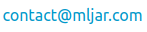The `mljar-supervised` is provided with MIT license.

# MLJAR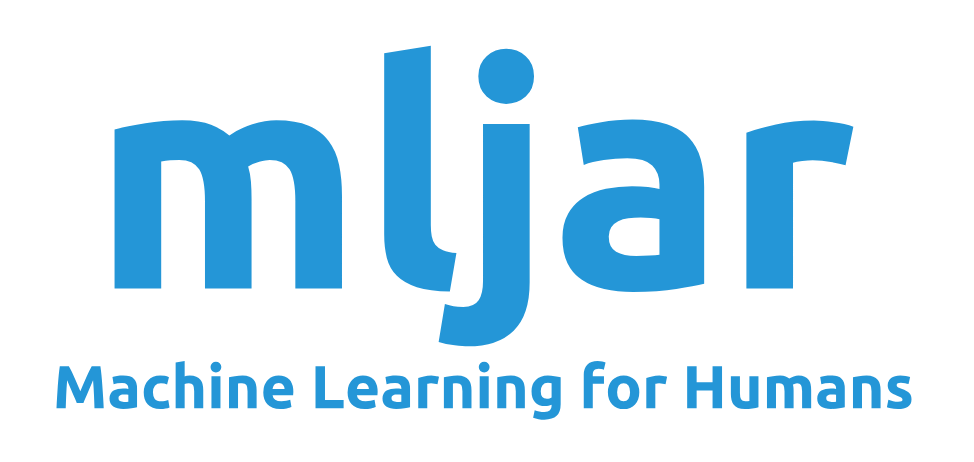The `mljar-supervised` is an open-source project created by MLJAR. We care about ease of use in the Machine Learning. The mljar.com provides a beautiful and simple user interface for building machine learning models.

Alternatives To Mljar Supervised
Select To Compare

Alternative Project Comparisons
Related Awesome Lists

Get A Weekly Email With Trending Projects For These Topics
No Spam. Unsubscribe easily at any time.
Python (889,550
Learning (75,673
Machine Learning (40,873
Ml (40,873
Markdown (30,240
Neural Network (16,105
Data Science (11,425
Plot (9,955
Scikit Learn (2,537
Xgboost (1,737
Decision Trees (1,469
Random Forest (1,352
Automl (834
Feature Engineering (699
Lightgbm (638
Hyperparameter Optimization (393
Catboost (104
Automated Machine Learning (90
Hyperparameters (76
Shap (68
Automatic Machine Learning (17
Mljar (5